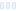# Instant Formula

## Probiotic, Powder, with Iron - Wegmans

Main info:

Instant Formula
Probiotic, Powder, with Iron - Wegmans
5 oz
100.0 Calories
10.9 g
5.3 g
2.1 g

0 g
0 mg
0 g
27.0 mg
0 g
0 g

Percent calories from...
Nutrition Facts
For a Serving Size of (g)
How many calories are in Instant Formula? Amount of calories in Instant Formula: Calories Calories from Fat (%)
% Daily Value *
How much fat is in Instant Formula? Amount of fat in Instant Formula: Total Fat
How much sodium is in Instant Formula? Amount of sodium in Instant Formula: Sodium
How much potassium is in Instant Formula? Amount of potassium in Instant Formula: Potassium
How many carbs are in Instant Formula? Amount of carbs in Instant Formula: Carbohydrates
How many net carbs are in Instant Formula? Amount of net carbs in Instant Formula: Net carbs
How much fiber is in Instant Formula? Amount of fiber in Instant Formula: Fiber
How much protein is in Instant Formula? Amount of protein in Instant Formula: Protein
Vitamins and minerals
How much Vitamin A is in Instant Formula? Amount of Vitamin A in Instant Formula: Vitamin A
How much Vitamin C is in Instant Formula? Amount of Vitamin C in Instant Formula: Vitamin C
How much Calcium is in Instant Formula? Amount of Calcium in Instant Formula: Calcium
How much Iron is in Instant Formula? Amount of Iron in Instant Formula: Iron
Fatty acids
Amino acids
* The Percent Daily Values are based on a 2,000 calorie diet, so your values may change depending on your calorie needs.Loading similar foods...
Note: Any items purchased after clicking our Amazon buttons will give us a little referral bonus. If you do click them, thank you!Be cool

- Zen orangeI never skip arm day

- Buff broccoli